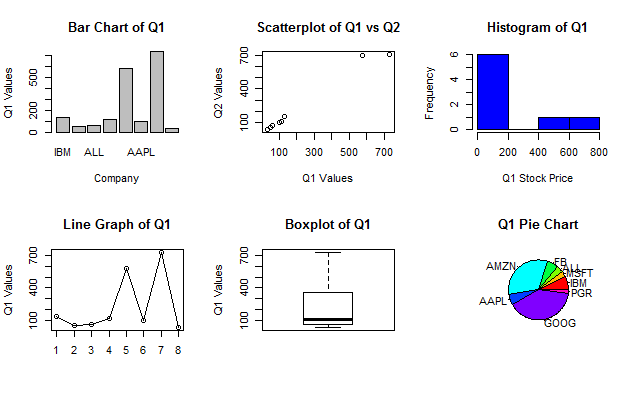# Creating Graphical Displays

### Bar Chart

A bar chart has one bar for each x-value, and counts the number of times that value appears. The basic syntax for a bar chart is: `barplot(height, names.arg, main = "Plot Title", "x-axis label", ylab = "y-axis label")`

Argument meanings:

• height: the respective heights of each bar
• names.arg: character vector; gives the names that correspond to each respective bar
• xlab: x-axis label
• ylab: y-axis label

In the example shown below, the variable xnames is first created and holds the labels that will be shown below each bar. This is done so that the code inside `barplot()` is more organized and easier to understand.

``````Q1 <- c(134.04, 53.23, 63.39, 116.89, 577.72, 100.22, 728.56, 32.47)
xnames <- c("IBM", "MSFT", "ALL", "FB", "AMZN", "AAPL", "GOOG", "PGR")
barplot(height = Q1, names.arg = xnames, main = "Quarter 1 Values", xlab = "Company", ylab = "Q1 Values")``````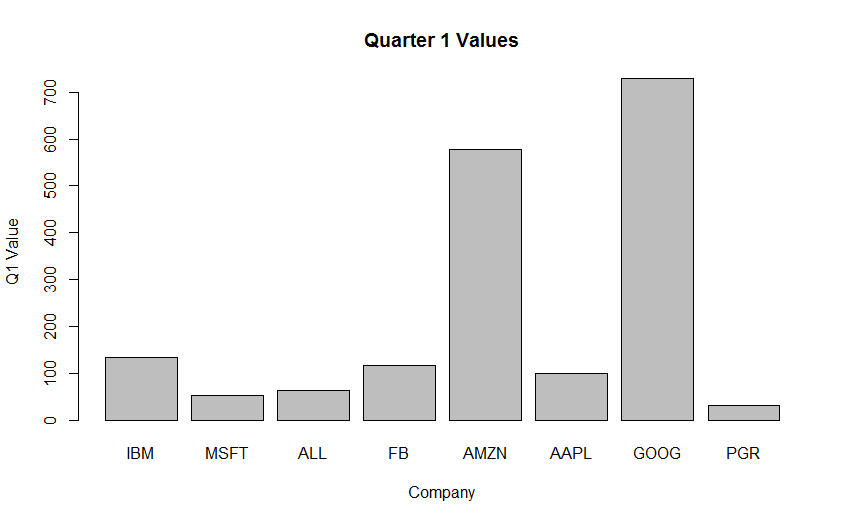### Scatterplot

A scatterplot is used to show how data points are spread out over a certain range of values. The basic syntax of a scatterplot is: `plot(x, y, main = "Plot Title", xlab = "x-axis label", ylab = "y-axis label")`.

Argument meanings:

• x: The x values along the horizontal axis
• y: The y values for each corresponding x value
• main: The main title of the plot and will be located above the plot itself
• xlab: x-axis label
• ylab: y-axis label
``````Q1 <- c(134.04, 53.23, 63.39, 116.89, 577.72, 100.22, 728.56, 32.47)
Q2 <- c(150.02, 51.23, 67.29, 111.08, 699.33, 96.21, 706.94, 31.13)
plot(x = Q1, y = Q2, main = "Q1 vs Q2", xlab = "Q1 Price", ylab = "Q2 Price")``````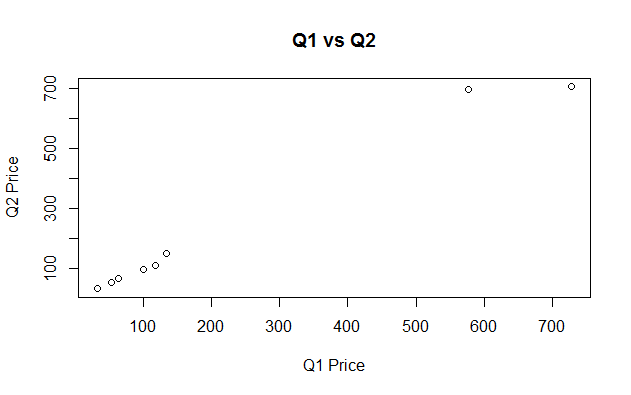Note: The variable num was made to have values from 1 to 8 instead of the company names. If the company names had been used instead of the numbers, an error would have been given that the x values should be numeric. If the x values were actually values, then you would simply provide the numeric vector instead.

### Histogram

A histogram is similar to a bar chart, but slightly different; a bar chart is used to measure categorical data (number of males vs. number of females, how many prefer dogs over cats, etc.), but a histogram is used to measure the frequency of a certain value within a dataset; this makes it useful at getting a quick look at rough distributions and notable factors in the data; the height of the bar represents the frequency of that range, or how many x-values fall within that bin.

It is important to note that bin sizes, i.e. the range where certain values fall into a given bar, are very important and can have a significant impact on the shape of the histogram, so they shouldn't be too big or too small. `hist(x, col, xlab, main)`

Argument meanings:

• x: The data that is going to be used
• col: The color of the bars
• xlab: The name along the x axis
• main: The name of the entire plot
``````Q1 <- c(134.04, 53.23, 63.39, 116.89, 577.72, 100.22, 728.56, 32.47)
hist(Q1, col = "blue", xlab = "Q1 Values", main = "Histogram of Q1")  ``````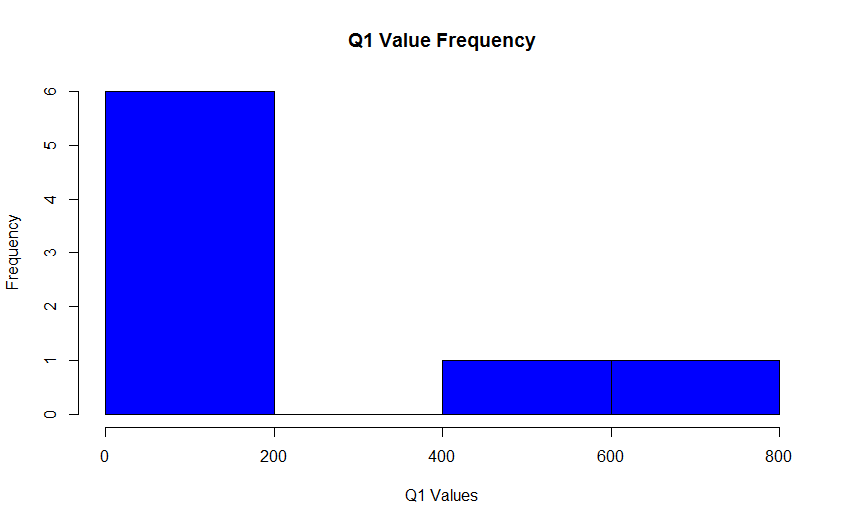### Line Graph

A line graph looks somewhat similar to a scatterplot, although it is actually more like a bar plot in that it has a series of x-values with one y-value each. It is traditionally used to show change over time, but is sometimes used in other ways as well.

A line graph is made by adding the following to the code for a scatterplot: `lines(x, y)`

As mentioned above, each x value corresponds to exactly one y value.

``````y <- c(134.04, 53.23, 63.39, 116.89, 577.72, 100.22, 728.56, 32.47)
plot(1:8, y, main = "Line Graph of Q1", xlab = "x", ylab = "y")
lines(1:8, Q1)``````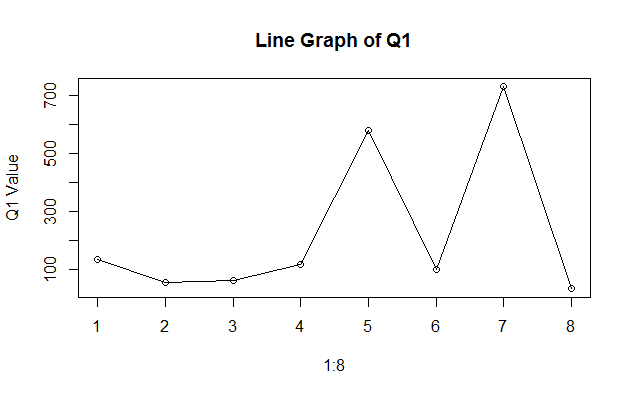Note: An alternative to the method above is to leave out the `lines()` function and instead add the `type =` argument to the plot function as follows:

• type = "b" will add points and lines to the plot, and look exactly like what is shown above.
• type = "l" adds just the lines to the plot, with the points themselves not shown.

Important: Notice that the x values are sorted from least to greatest in this example. That is because these functions will draw the lines between points in the order they are entered, so make sure your points are sorted from properly when you enter them into the function if you want to have lines that don't intersect. Again, this applies to both the `lines()` function and the `type =` argument in `plot()`.

### Boxplot

A boxplot is used to show the range of values in a dataset. The basic syntax is shown below: `boxplot(x = dataset, main = "Title", ylab = "Label")`

Argument meanings:

• x: The data to be used in the boxplot
• main: The name of the whole plot
• ylab: The name of the y axis
``````Q1 <- c(134.04, 53.23, 63.39, 116.89, 577.72, 100.22, 728.56, 32.47)
boxplot(Q1, main = "Q1 Value Boxplot", ylab = "Q1 Values")``````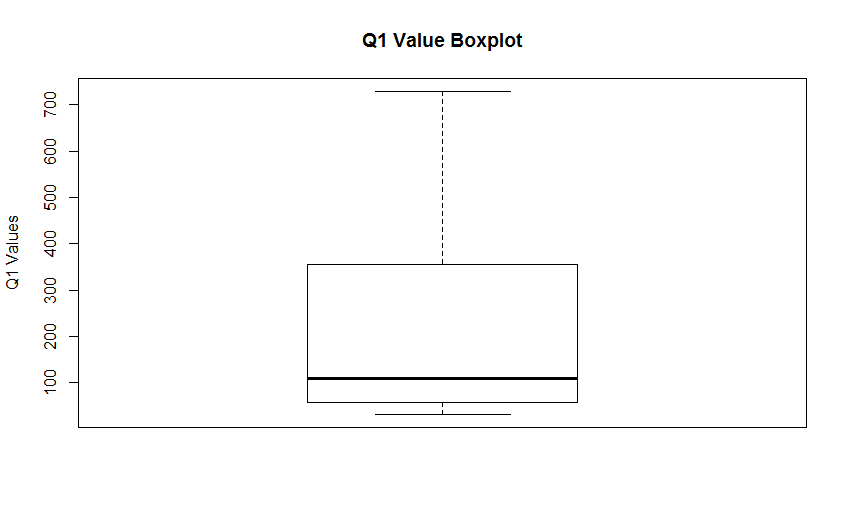### Pie Chart

A pie chart is used to show visually the percentage of the whole data a certain value makes up. The basic syntax is shown below: `pie(x = dataset, labels = "names", col = colors, main = "Title"`

Parameter meanings:

• x: The data to be used in the chart
• labels: The names for each split part of the pie chart
• col: The colors of the parts in the chart
• main: The name for the entire chart
``pie(x=Q1, labels=xnames, col=rainbow(length(xnames)), main="Q1 Variable Pie Chart")``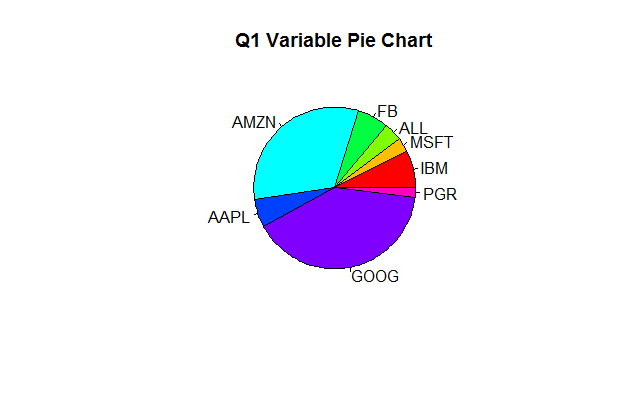### Subplots

A subplot is used when you want to see a number of plots side by side. The syntax is shown below: `par(mfrow = c(x, y))`

• mfrow: makes an x-by-y table of plots

This code will allow a matrix of plots to be shown with 2 rows and 3 columns for a total of 6 plots.

``````Q1 <- c(134.04, 53.23, 63.39, 116.89, 577.72, 100.22, 728.56, 32.47)
Q2 <- c(150.02, 51.23, 67.29, 111.08, 699.33, 96.21, 706.94, 31.13)
xnames <- c("IBM", "MSFT", "ALL", "FB", "AMZN", "AAPL", "GOOG", "PGR")

par(mfrow = c(2, 3))
barplot(Q1, names.arg = xnames, main = "Bar Chart of Q1", xlab = "Company", ylab = "Q1 Values")
plot(Q1, Q2, main = "Scatterplot of Q1 vs Q2", xlab = "Q1 Values", ylab = "Q2 Values")
hist(Q1, col = "blue", xlab = "Q1 Stock Price", main = "Histogram of Q1")
plot(1:8, Q1, main = "Line Graph of Q1", xlab = "", ylab = "Q1 Values")
lines(1:8, Q1)
boxplot(Q1, main = "Boxplot of Q1", ylab = "Q1 Values")
pie(Q1, labels = xnames, col = rainbow(length(xnames)),  main = "Q1 Pie Chart")``````